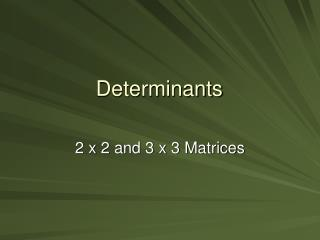DownloadDownload PresentationDeterminants

# Determinants

Télécharger la présentation## Determinants

- - - - - - - - - - - - - - - - - - - - - - - - - - - E N D - - - - - - - - - - - - - - - - - - - - - - - - - - -
##### Presentation Transcript

1. Determinants 2 x 2 and 3 x 3 Matrices

2. 1 3 -½ 0 -3 8 ¼ 2 0 -¾ 4 180 11 Matrices Note that Matrix is the singular form, matrices is the plural form! • A matrix is an array of numbers that are arranged in rows and columns. • A matrix is “square” if it has the same number of rows as columns. • We will consider only 2x2 and 3x3 square matrices

3. Note the difference in the matrix and the determinant of the matrix! Determinants • Every square matrix has a determinant. • The determinant of a matrix is a number. • We will consider the determinants only of 2x2 and 3x3 matrices.

4. Determinant of a 2x2 matrix

5. Determinant of a 3x3 matrix Imagine crossing out the first row. And the first column. Now take the double-crossed element. . . And multiply it by the determinant of the remaining 2x2 matrix

6. Determinant of a 3x3 matrix Now keep the first row crossed. Cross out the second column. • Now take the negative of the double-crossed element. • And multiply it by the determinant of the remaining 2x2 matrix. • Add it to the previous result.

7. Determinant of a 3x3 matrix Finally, cross out first row and last column. • Now take the double-crossed element. • Multiply it by the determinant of the remaining 2x2 matrix. • Then add it to the previous piece.